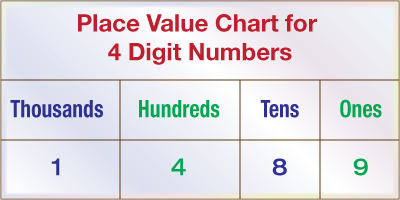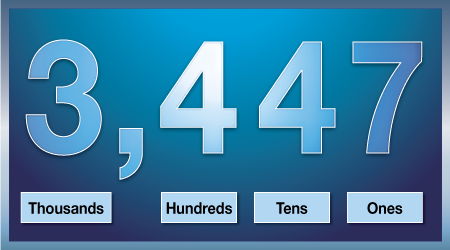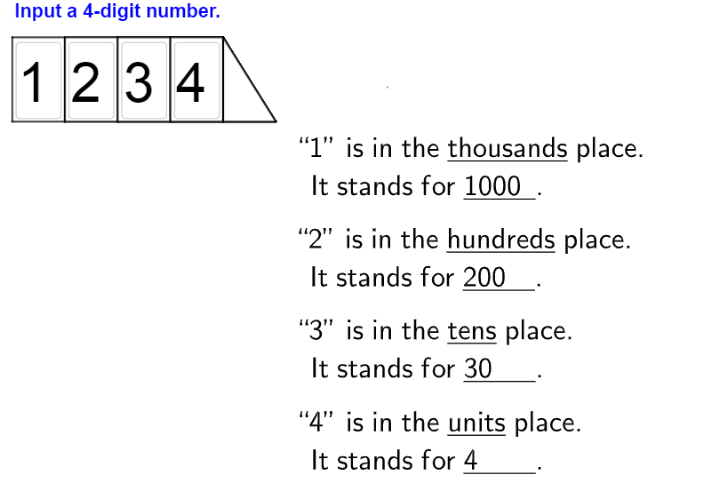# Numbers up to 4-Digits

Go back to  'Numbers and Place values'

## Introduction to Numbers up to $$4$$-Digits

When we multiply a unit with ten, we get a two digit number. For example, $$2$$ multiplied by $$10$$ gives $$20.$$ For $$3$$ digit numbers it’s \begin{align} 10 \times 10 = 100. \end{align}

What happens when we multiply a unit digit with $$1000?$$ In such a number, the following place values are covered:Each of the above place values would have a number, and so you would get a number with $$4$$ digits. After $$999,$$ the first $$1$$ digit number begins with $$1000.$$ The next $$9000$$ numbers are all $$4$$ digit numbers, for example $$3469, 9210, 2583$$ etc.

## The Big Idea: Numbers up to $$4$$-Digits

### A simple idea: The Place Value of Numbers

When we covered $$3$$-digit numbers, we saw that the hundreds place value was the units place multiplied by a hundred. Like you’ve already read, for four digit numbers a new place value is introduced which is the units place multiplied by $$1000.$$

It’s not that hard to visualise actually, take any $$3$$ digit and multiply it with $$10,$$ and there you go! A four digit number!### How To Decompose $$4$$-digit numbers

We already saw that a $$4$$ digit number has the following place values – thousands, hundreds, tens and units. That is why the multiplier to be used for each of the digits of a $$4$$-digit number are $$1000, 100, 10$$ and $$1$$ respectively.

Let us examine the number $$1729.$$ We can decompose the number as

\begin{align} { 1729 = ( 1 \times 1000 ) + ( 7 \times 100 ) + ( 2 \times 10 ) + ( 9 \times 1 ) = 1000 + 700 + 20 + 9 = } { 1729 } \end{align}

Consider the number $$1792, 1279$$ and $$2179$$ as well. And notice the values of the same digit in each of these numbers.

\begin{align} &{ 1729 - 2 \text { has ten's place and a place value of } 20 } \\ &{ 1792 - 2 \text { has unit's place and a place value of } 2 } \\ &{ 1279 - 2 \text { has hundred's place and a place value of } 200 } \\& { 2179 - 2 \text { has thousand's place and a place value of } 2000 } \end{align}

What this tells us is that the value of a digit is not the only thing that determines its place value in a $$4$$-digit number. In $$1729,$$ for instance, the number $$1$$ has a lower value than the number $$2,$$ but in terms of place value, $$1$$ has a place value of $$1000$$ (being the $$4^{\text{th}}$$ digit from right) and $$2$$ has a place value of $$10$$ (being the second digit from right).

You can try some more $$4$$-digit numbers here to see how they can be decomposed into their place values.### Commas in $$4$$-digit numbers

In every $$4$$-digit number, the $$4^{\text{th}}$$ digit on the extreme left represents the thousand’s place. It is a common convention to apply a comma between the $$4^{\text{th}}$$ and $$3^{\text{rd}}$$ digit (thousand’s place and hundred’s place). So, while the $$3$$-digit number $$374$$ is written simply as $$374,$$ the $$4$$-digit number $$4539$$ is written as $$4,539.$$

There is no mathematical reason for doing this. The $$4$$-digit number is longer than a $$3$$-digit or $$2$$-digit number, and the comma only helps to make the number more readable.

## How is it important?

### The Amazing Kaprekar’s Routine

As per this amazing law, there is a magic number $$6174.$$ Let us see how we get it. These are the steps to be followed.

1. Arrange any $$4$$-digit number in ascending order of digit and descending order of digits.
2. This was you get two distinct $$4$$-digit numbers.
3. Find out the difference between the two numbers.
4. Repeat the above $$3$$ steps on this number.
5. You will reach $$6174$$ in at most $$7$$ iterations.

Doesn’t it sound amazing enough for us to try it out? Ok, so let’s say I choose the number $$2873.$$ The digits arranged in ascending order give us $$2378,$$ and $$8732.$$

What is $$8732 - 2378?$$ The answer is $$6354.$$

Again this gives two numbers $$3456$$ and $$6543.$$

What is $$6543 - 3456?$$ The answer is $$3087.$$

After that, $$8730 - 0378 = 8325.$$

Finally $$8532 - 2358 = 6174!!$$

Whatever $$4$$ digit number you choose, you will reach $$6174$$ within at most $$7$$ iterations.

Now what happens after $$6174$$ is reached? It gives the two numbers $$7641$$ and $$1467,$$ and the difference between these two numbers is $$6174$$ again, so this sequence keeps getting repeated ad infinitum. This interesting phenomenon was first observed and formalized by the Indian mathematician Kaprekar. That is why this sequence is known as the Kaprekar routine.

### An Interesting Story to End

Ramanujam was one the greatest Indian mathematicians. One day he fell ill, and his friend Hardy came to visit him. Hardy decided to tease his mathematician friend by saying that the cab I came in had a very uninteresting number plate – $$1729.$$ Even though he was not keeping well, Ramanujam’s sharp brain was still working, and he immediately retorted that $$1729$$ was actually a very interesting number, because it was the smallest number which could be expressed as the sum of two cubes in two different and unique ways!! If this was how sharp he was when unwell, imagine what his brain was capable of when functioning fully. In honor of this incident, the number $$1729$$ has been referred to as the Hardy – Ramanujam number.